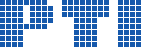Polish Information Processing Society# Annals of Computer Science and Information Systems, Volume 11

## Is there a computable upper bound on the heights of rational solutions of a Diophantine equation with a finite number of solutions?

### Krzysztof Molenda, Agnieszka Peszek, Maciej Sporysz, Apoloniusz Tyszka

Citation: Proceedings of the 2017 Federated Conference on Computer Science and Information Systems, M. Ganzha, L. Maciaszek, M. Paprzycki (eds). ACSIS, Vol. 11, pages 249258 ()

Full text

Abstract. The height of a rational number p/q is denoted by h(p/q) and equals max(|p|,|q|) provided p/q is written in lowest terms. The height of a rational tuple (x\_1,...,x\_n) is denoted by h(x\_1,...,x\_n) and equals max(h(x\_1),...,h(x\_n)). Let G\_n={x\_i+1=x\_k: i,k \in {1,...,n}} \cup {x\_i \cdot x\_j=x\_k: i,j,k \in {1,...,n}}. Let f(1)=1, and let f(n+1)=2^(2^(f(n))) for every positive integer n. We conjecture: (1) if a system S \subseteq G\_n has only finitely many solutions in rationals x\_1,...,x\_n, then each such solution (x\_1,...,x\_n) satisfies h(x\_1,...,x\_n) \leq {1 (if n=1), 2^(2^(n-2)) (if n> 1)}; (2) if a system S \subseteq G\_n has only finitely many solutions in non-negative rationals x\_1,...,x\_n, then each such solution (x\_1,...,x\_n) satisfies h(x\_1,...,x\_n) \leq f(2n). We prove: (1) both conjectures imply that there exists an algorithm which takes as input a Diophantine equation, returns an integer, and this integer is greater than the heights of rational solutions, if the solution set is finite; (2) both conjectures imply that the question whether or not a given Diophantine equation has only finitely many rational solutions is decidable by a single query to an oracle that decides whether or not a given Diophantine equation has a rational solution.

### References

1. A. Bremner, Positively prodigious powers or how Dudeney done it? Math. Mag. 84 (2011), no. 2, 120–125, http://dx.doi.org/10.4169/math.mag.84.2.120.
2. M. Davis, Representation theorems for recursively enumerable sets and a conjecture related to Poonen’s large subring of Q, J. Math. Sci. (N. Y.) 171 (2010), no. 6, 728–730. http://dx.doi.org/10.1007/s10958-010-0176-7.
3. F. G. Dorais, Can the twin prime problem be solved with a single use of a halting oracle? July 23, 2011, http://mathoverflow.net/questions/71050.
4. H. Friedman, Complexity of statements, April 20, 1998, http://www.cs.nyu.edu/pipermail/fom/1998-April/001843.html.
5. T. Gowers, J. Barrow-Green, I. Leader (eds.), The Princeton companion to mathematics, Princeton University Press, Princeton, 2008.
6. M. Kim, On relative computability for curves, Asia Pac. Math. Newsl. 3 (2013), no. 2, 16–20, http://www.asiapacific-mathnews.com/03/0302/0016 0020.pdf.
7. M. Mignotte and A. Pethő, On the Diophantine equation xp − x = yq − y, Publ. Mat. 43 (1999), no. 1, 207–216.
8. J. Robinson, Definability and decision problems in arithmetic, J. Symbolic Logic 14 (1949), 98–114; reprinted in: The collected works of Julia Robinson (ed. S. Feferman), Amer. Math. Soc., Providence, RI, 1996, 7–23.
9. W. Sierpiński, Elementary theory of numbers, 2nd ed. (ed. A. Schinzel), PWN (Polish Scientific Publishers) and North-Holland, Warsaw-Amsterdam, 1987.
10. S. Siksek, Chabauty and the Mordell–Weil Sieve, in: Advances on Superelliptic Curves and Their Applications (eds. L. Beshaj, T. Shaska, E. Zhupa), 194–224, IOS Press, Amsterdam, 2015, http://dx.doi.org/10.3233/978-1-61499-520-3-194.
11. C. Smoryński, A note on the number of zeros of polynomials and exponential polynomials, J. Symbolic Logic 42 (1977), no. 1, 99–106.
12. A. Tyszka, Conjecturally computable functions which unconditionally do not have any finite-fold Diophantine representation, Inform. Process. Lett. 113 (2013), no. 19–21, 719–722, http://dx.doi.org/10.1016/j.ipl.2013.07.004.
13. A. Tyszka, A common approach to Brocard’s problem, Landau’s problem, and the twin prime problem, March 28, 2017, http://arxiv.org/abs/1506.08655v21.